you are here->home->Physics->Class 11->Friction
Friction

# Objectives

1.    To study the relationship between force of limiting friction and normal reaction.
2.    To find the co-efficient of friction between a block and a horizontal surface.

# Theory

When one body makes an attempt to slide over another body, an opposing force called the force of friction arises as a reaction to the applied force, and acts in the opposite direction.

Static friction is the type of friction that exists when two objects touch each other at rest. It has a limiting value called limiting friction, which is equal to the least force required to move the body from rest. When the external force F is increased, a stage comes when the body is just at the verge of moving. At this stage, the force of friction is at the maximum, and is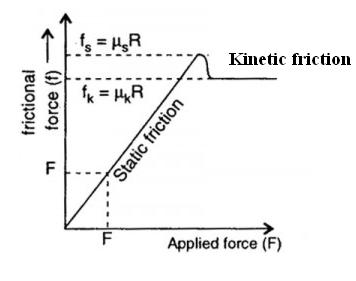called limiting friction.

It is found experimentally that limiting frictional force,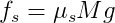whereis called the coefficient of static friction. It is a dimensionless constant, but depends on the nature of surfaces in contact.

When the applied force, F, is increased further (beyond the limiting frictional force), the body begins to move, then the force opposing the motion is called the kinetic or sliding friction. The kinetic friction is less than the limiting friction. The force of kinetic friction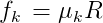where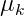is called the coefficient of kinetic friction.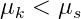### The Laws of Static Friction are:

i.  The magnitude of limiting friction depends upon the nature and state of polish of the two surfaces in contact.
ii. The magnitude of limiting friction is independent of area and shape of surfaces in contact as long as the normal reaction    remains the same.
iii. The magnitude of limiting friction 'F' is directly proportional to normal reaction 'R' between the two surfaces in contact.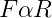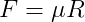where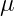is called the coefficient of friction which is a measure of friction based on the type of material that are in contact.The coefficient of friction,A graph can be drawn with normal reaction, R along X axis and limiting friction, F along Y axis and it is a straight line.

# Learning Outcomes:

• Students understand underlying concepts of friction.
• They learn about limiting friction, normal reaction and its relationship.
• They understand coefficient of friction.

Cite this Simulator: